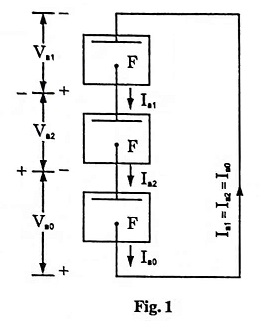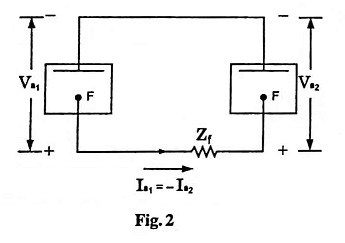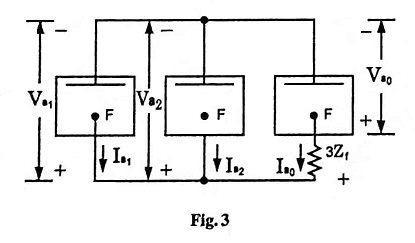## Unsymmetrical Fault Analysis Interview Questions and Answers:

1. Draw the sequence network for single line-to-ground fault without fault impedance.

Ans.2. Draw the sequence network connections corresponding to L-L fault at a bus.

Ans.3. How are sequence networks interconnected in the case of L-L-G fault ?

Ans. Three symmetrical component sequence networks are connected in parallel for a bold L-L-G fault, as shown in the fig. 34. What are the different types of faults which occur in a power system ?

Ans. The most common and dangerous faults that occur in a power system are the short circuit or shunt faults such as L-G, L-L, L-L-G, L-L-L and L-L-L-G faults. The other type of faults that occur in a power system are series type such as one conductor open fault and two conductor open fault.

5. What are unsymmetrical faults ?

Ans. The faults giving rise to unsymmetrical currents i.e., currents differing in magnitude and phases in the three phases of the power system are known as unsymmetrical faults. Unsymmetrical faults may also be defined as the faults involving one or two phases (not involving 3 phases) such as L-G, L-L, L-L-G faults.

6. Distinguish between symmetrical and unsymmetrical faults.

Ans. A fault involving all the three phases is known as symmetrical (balanced) fault while one involving only one or two phases is known as unsymmetrical fault. Single line-to-ground (L-G), line-to-line (L-L), double line-to-ground (L-L-G) faults are unsymmetrical (unbalanced) faults. Majority of the faults are unsymmetrical ones.

7. For a fault at a given location rank the various faults in the order of severity.

Ans. The faults in increasing order of severity are L-G, L-L, L-L-G and symmetrical three-phase faults (L-L-L or L-L-L-G).

8. Name the faults which do not have zero sequence currents flowing.

Ans. In line-to-line faults and symmetrical faults (L-L-L or L-L-L-G faults) no zero sequence current flows.

9. Name the fault in which all the three sequence currents are present and are equal to each other.

Ans. In L-G fault all the three sequence currents are present and are equal to each other.

10. Which of the faults would cause a greater electromagnetic interference between the power line and a nearby communication line ?

Ans. Single phase-to-ground (L-G) fault, due to heavy unbalancing of line currents, causes greater electromagnetic interference between the power line and a nearby communication line.

Scroll to Top